• +91 9971497814
• info@interviewmaterial.com

# RD Chapter 23- The Straight Lines Ex-23.9 Interview Questions Answers

### Related Subjects

Question 1 :

Reduce the equation √3x + y + 2 = 0 to:
(i) slope – intercept form and find slope and y – intercept;
(ii) Intercept form and find intercept on the axes
(iii) The normal form and find p and α.

(i) Given:

√3x + y + 2 = 0

y = – √3x – 2

This is the slopeintercept form of the given line.

The slope = – √3and y – intercept = -2

(ii) Given:

√3x + y + 2 = 0

√3x + y = -2

Divide both sides by-2, we get

√3x/-2 + y/-2 = 1

The intercept form ofthe given line. Here, x – intercept = – 2/√3 and y – intercept = -2

(iii) Given:

√3x + y + 2 = 0

-√3x – y = 2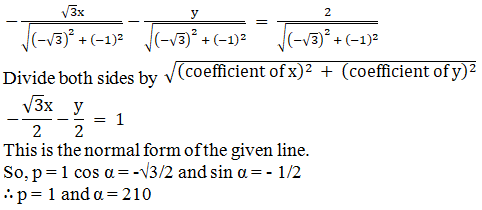Question 2 :
Reduce the following equations to the normal form and find p and α in each case:
(i) x + √3y – 4 = 0
(ii) x + y + √2 = 0

(i) x + √3y – 4 = 0

x + √3y = 4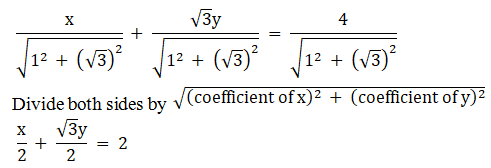The normal form of thegiven line, where p = 2, cos α = 1/2 and sin α = √3/2

p = 2 and α = π/3

(ii) x + y + √2 = 0

-x – y = √2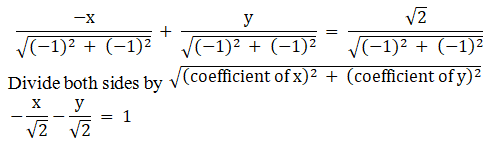The normal form of thegiven line, where p = 1, cos α = -1/√2 and sin α = -1/√2

p = 1 and α = 225o

Question 3 : Put the equation x/a + y/b = 1 the slope intercept form and find its slope and y – intercept.

Given: the equationis x/a + y/b = 1

We know that,

General equation ofline y = mx + c.

bx + ay = ab

ay = – bx + ab

y = -bx/a + b

The slope interceptform of the given line.

Slope = – b/aand y – intercept = b

Question 4 : Reduce the lines 3x – 4y + 4 = 0 and 2x + 4y – 5 = 0 to the normal form and hence find which line is nearer to the origin.

The normal forms ofthe lines 3x − 4y + 4 = 0 and 2x + 4y − 5 = 0.

Let us find, in givennormal form of a line, which is nearer to the origin.

-3x + 4y = 4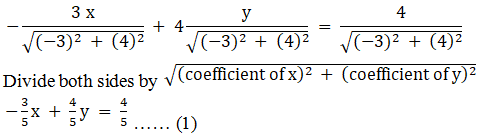Now 2x + 4y = – 5

-2x – 4y = 5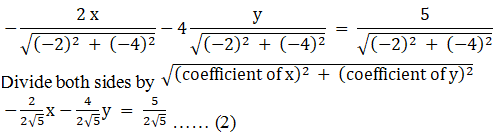From equations (1) and(2):

45 < 525

The line 3x − 4y+ 4 = 0 is nearer to the origin.

Question 5 : Show that the origin is equidistant from the lines 4x + 3y + 10 = 0; 5x – 12y + 26 = 0 and 7x + 24y = 50.

Given:

The lines 4x + 3y + 10= 0; 5x – 12y + 26 = 0 and 7x + 24y = 50.

We need to prove that,the origin is equidistant from the lines 4x + 3y + 10 = 0; 5x – 12y + 26 = 0and 7x + 24y = 50.

Let us write down thenormal forms of the given lines.

First line: 4x + 3y +10 = 0

-4x – 3y = 10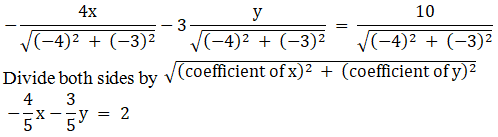So, p = 2

Second line: 5x − 12y+ 26 = 0

-5x + 12y = 26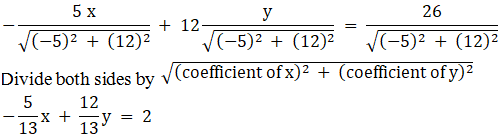So, p = 2

Third line: 7x + 24y =50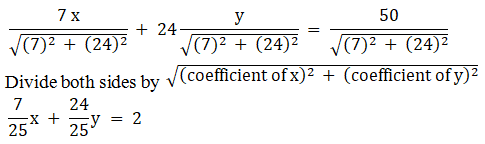So, p = 2

The origin isequidistant from the given lines.

Todays Deals### RD Chapter 23- The Straight Lines Ex-23.9 Contributorskrishan

Name:
Email:

# Latest News# 9000 interview questions in different categories